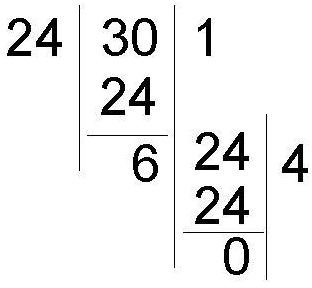# Two Methods of Finding the Greatest Common Factor: Math Help

Page content

## What is the GCF?

As the name indicates, Greatest Common Factor (GCF) is the common and largest factor of the given set of numbers (for which GCF is to be calculated). To find out the GCF,

1. Factorize the numbers.
2. Compare the factors.
3. Find all the common prime factors and multiply them.

## Example

The following example uses 24 and 30.

1. Factorize both numbers and write them on board:

• 24 = 2 x 2 x 2 x 3

• 30 = 3 x 2 x 5

2. Find the common factors of the two numbers.

• 2 and 3.
3. Because both 30 and 24 have 2 and 3 as a prime factor, multiply these two numbers together.

4. Write the product of 2 and 3 as 6. This is the GCF of 24 and 30.

## Another Method

Please understand another method of finding the Greatest Common Factor:

1. Take the smallest number (say A) among the numbers for which GCF needs to find out.
2. Divide any one of the other number (say B) by this number (A).
3. Find reminder (say C) of the division.
4. Divide the divider (A) of the earlier division by the remainder (C).
5. Find remainder (say D).
6. Divide C by D.
7. Repeat step 6 until there is no remainder.
8. The last divider for which there is no remainder is the GCF of the two numbers.

See the figure below for better clarity of the process.

If you have more than two numbers, then first find out the GCF of any two numbers and use that GCF to divide any one of the remaining numbers and so on to find final GCF.

Once you are clear with this method of finding GCF, explain it by taking example of 24 and 30. Like below:As you can see, both methods produce the same result.